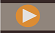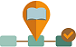## Enabling New Capital Investment

Enables new capital investments for fixed assets and optionally allows you to plan for lease assets (for example, vehicles) or intangibles (for example, copyrights). Select Named Assets to add the names of assets to plan at the detail level, and specify the likely number of named assets that you would add in a planning cycle.

If you are going to use Named Assets, you must select it the first time you enable Capital. You can’t come back later to enable it. You can, however, come back later to increment the number of named assets.

IFRS16 in Lease Asset Planning

If you are performing lease asset planning, you can incrementally enable IFRS16 support by selecting IFRS16 – Standard under Lease Assets.

When you enable IFRS16-Standard, the following changes occur:

• The Calculate Leased Assets rule is updated to use the new standards for calculating lease assets. (If you don’t enable IFRS16-Standard, the rule is not updated.)

• Two new members are available in Depreciation & Amortization AssumptionsLow Value Lease Term (in Months) and Low Value Lease Amount. For low value assets, you can choose to write off the asset on the P&L rather than carrying the asset value on the Balance Sheet. For example, lease assets of 12 months lease term or \$5000 value are considered low value assets, however you can change the values for each asset type. Lease assets that meet the low value thresholds you entered are calculated using the IFRS16 standard for low value assets, and are charged off on the P&L rather than being treated like other assets.

• Payment frequency is taken into account when determining if a lease asset is a low value asset. The value of the asset is calculated as (`lease payment` x `payment frequency`). If the calculated value is less than the Low Value Lease Amount, the asset is considered to be a low value lease asset.

For example, if the Low Value Lease Amount is \$5000, and a lease asset has a payment of \$500 with a monthly payment frequency, the lease asset value is calculated as \$500 x 12 months = \$6000. Because \$6000 is higher than the Low Value Lease Amount of \$5000, this lease asset is not considered to be a low value asset. However, if this same asset has a semi-annual payment frequency, the lease asset value is calculated as \$500 x 2 = \$1000, which is less than the Low Value Lease Amount of \$5,000, and is considered to be a low value asset.

• A new account - Low Value Override is created. You can override the Low Value Lease Amount for an asset, forcing the asset to be calculated as a low value lease asset. The Low Value Override column is added on the Lease Asset Planning form. By default, the value for Low Value Override for each asset is No. If you change it to Yes for an asset, the asset is calculated as a low value asset regardless of the lease asset value.
• A new asset account, Index Rate, is added. You can set an Index Rate when you create a new lease asset.

• When you set an Index Rate, lease amounts increase for inflation based on the Index Rate Basis (annual or according to the payment frequency, depending on what you select).
• The Calculate Leased Assets business rule takes the index rate and index rate basis into account when calculating the NPV of an asset. If you don’t set the index rate, the lease payments are the same for each period.
• For existing assets, you can enter the Index Rate on the New Leased Assets form in Investments, Leased Asset Planning.

• A new account, Index Rate Basis, is added.
• A new Smart List is available for Index Rate Basis with entries for Annual and Payment Frequency.

• Annual—Applies inflation annually irrespective of the frequency of payments.
• Payment Frequency—Applies inflation based on the payment frequency.
• When you add a new lease asset, in Add Lease Asset, select Annual or Payment Frequency for Index Rate Basis. By default, Index Rate Basis for new leased assets is Annual.

• You can change the Index Rate Basis at any time on the Lease Asset Planning form.

• Rent free periods, also known as a lease holiday, are available.
• A new form, Rent Free Periods, is available.
• A new account, OCX_LeaseRentFreePeriod, is available to capture whether a period is rent free.
• Rent free periods are based on periods, not a date range, based on the payment frequency for the lease (monthly, quarterly, yearly).

• After you add new lease assets, on the Rent Free Periods form, for each lease asset, select Yes for any periods that should be rent free.

• Rent free period lease payments are set to zero, and when you calculate the lease, values for present value and cash flow are calculated considering rent free periods.

• New rules are available:
• OCX_Free Rent Asset BeforeLoad. Attached to the Rent Free Period form to make all the cells read only and according to payment frequency only the corresponding cells are editable.
• OCX_Free Rent Period Spread. Attached to the Rent Free Period form to spread the quarter/semi annual/annual to months.
• Lease asset depreciation varies based on the ownership of the lease. If ownership is with the lessor, depreciation is charged for lease term or until the end of useful life of the asset - whichever is earlier. If ownership is with the lessee, depreciation is charged for useful life of the asset.

• You can plan using 12-month or 13-period calendars for lease assets.

• The alias for account OCX_Operating_Lease_Payments is changed from Operating Lease Payments to Lease Payments.

After enabling IFRS16 – Standard, enter Low Value Lease Term (in Months) and Low Value Lease Amount values in Depreciation & Amortization Assumptions. See Entering Capital Asset Assumptions.

VideoOverview: Lease Assets in Capital Planning for IFRS 16Lease Asset Planning with IFRS16